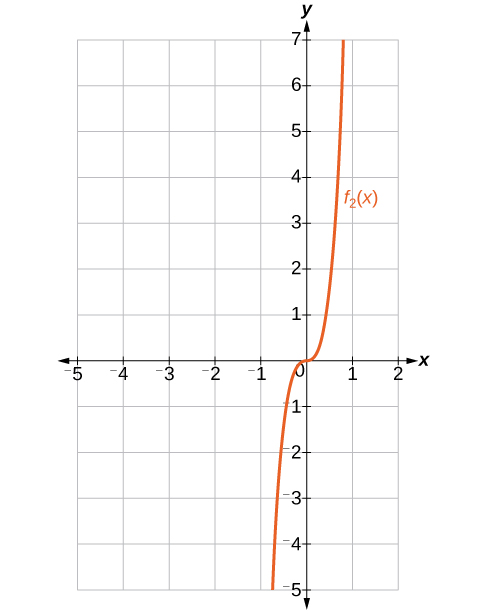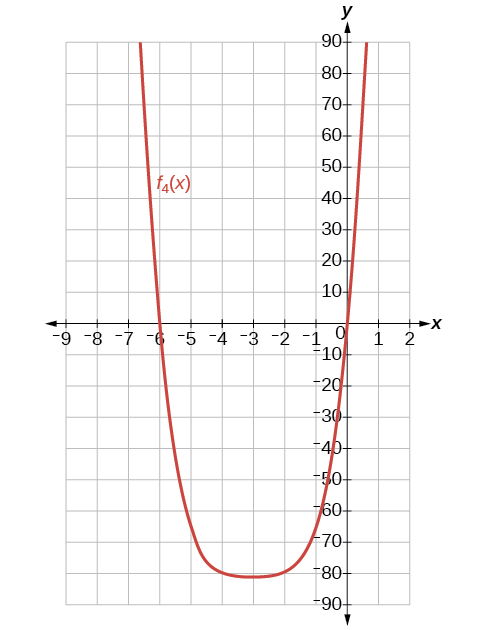# 13.6 Binomial theorem  (Page 3/6)

 Page 3 / 6

## Key equations

 Binomial Theorem ${\left(x+y\right)}^{n}=\sum _{k-0}^{n}\left(\begin{array}{c}n\\ k\end{array}\right){x}^{n-k}{y}^{k}$ $\left(r+1\right)th\text{\hspace{0.17em}}$ term of a binomial expansion $\left(\begin{array}{c}n\\ r\end{array}\right){x}^{n-r}{y}^{r}$

## Key concepts

• $\left(\begin{array}{c}n\\ r\end{array}\right)\text{\hspace{0.17em}}$ is called a binomial coefficient and is equal to $C\left(n,r\right).\text{\hspace{0.17em}}$ See [link] .
• The Binomial Theorem allows us to expand binomials without multiplying. See [link] .
• We can find a given term of a binomial expansion without fully expanding the binomial. See [link] .

## Verbal

What is a binomial coefficient, and how it is calculated?

A binomial coefficient is an alternative way of denoting the combination $\text{\hspace{0.17em}}C\left(n,r\right).\text{\hspace{0.17em}}$ It is defined as $\text{\hspace{0.17em}}\left(\begin{array}{c}n\\ r\end{array}\right)=\text{\hspace{0.17em}}C\left(n,r\right)\text{\hspace{0.17em}}=\frac{n!}{r!\left(n-r\right)!}.$

What role do binomial coefficients play in a binomial expansion? Are they restricted to any type of number?

What is the Binomial Theorem and what is its use?

The Binomial Theorem is defined as $\text{\hspace{0.17em}}{\left(x+y\right)}^{n}=\sum _{k=0}^{n}\left(\begin{array}{c}n\\ k\end{array}\right){x}^{n-k}{y}^{k}\text{\hspace{0.17em}}$ and can be used to expand any binomial.

When is it an advantage to use the Binomial Theorem? Explain.

## Algebraic

For the following exercises, evaluate the binomial coefficient.

$\left(\begin{array}{c}6\\ 2\end{array}\right)$

15

$\left(\begin{array}{c}5\\ 3\end{array}\right)$

$\left(\begin{array}{c}7\\ 4\end{array}\right)$

35

$\left(\begin{array}{c}9\\ 7\end{array}\right)$

$\left(\begin{array}{c}10\\ 9\end{array}\right)$

10

$\left(\begin{array}{c}25\\ 11\end{array}\right)$

$\left(\begin{array}{c}17\\ 6\end{array}\right)$

12,376

$\left(\begin{array}{c}200\\ 199\end{array}\right)$

For the following exercises, use the Binomial Theorem to expand each binomial.

${\left(4a-b\right)}^{3}$

$64{a}^{3}-48{a}^{2}b+12a{b}^{2}-{b}^{3}$

${\left(5a+2\right)}^{3}$

${\left(3a+2b\right)}^{3}$

$27{a}^{3}+54{a}^{2}b+36a{b}^{2}+8{b}^{3}$

${\left(2x+3y\right)}^{4}$

${\left(4x+2y\right)}^{5}$

$1024{x}^{5}+2560{x}^{4}y+2560{x}^{3}{y}^{2}+1280{x}^{2}{y}^{3}+320x{y}^{4}+32{y}^{5}$

${\left(3x-2y\right)}^{4}$

${\left(4x-3y\right)}^{5}$

$1024{x}^{5}-3840{x}^{4}y+5760{x}^{3}{y}^{2}-4320{x}^{2}{y}^{3}+1620x{y}^{4}-243{y}^{5}$

${\left(\frac{1}{x}+3y\right)}^{5}$

${\left({x}^{-1}+2{y}^{-1}\right)}^{4}$

$\frac{1}{{x}^{4}}+\frac{8}{{x}^{3}y}+\frac{24}{{x}^{2}{y}^{2}}+\frac{32}{x{y}^{3}}+\frac{16}{{y}^{4}}$

${\left(\sqrt{x}-\sqrt{y}\right)}^{5}$

For the following exercises, use the Binomial Theorem to write the first three terms of each binomial.

${\left(a+b\right)}^{17}$

${a}^{17}+17{a}^{16}b+136{a}^{15}{b}^{2}$

${\left(x-1\right)}^{18}$

${\left(a-2b\right)}^{15}$

${a}^{15}-30{a}^{14}b+420{a}^{13}{b}^{2}$

${\left(x-2y\right)}^{8}$

${\left(3a+b\right)}^{20}$

$3,486,784,401{a}^{20}+23,245,229,340{a}^{19}b+73,609,892,910{a}^{18}{b}^{2}$

${\left(2a+4b\right)}^{7}$

${\left({x}^{3}-\sqrt{y}\right)}^{8}$

${x}^{24}-8{x}^{21}\sqrt{y}+28{x}^{18}y$

For the following exercises, find the indicated term of each binomial without fully expanding the binomial.

The fourth term of $\text{\hspace{0.17em}}{\left(2x-3y\right)}^{4}$

The fourth term of $\text{\hspace{0.17em}}{\left(3x-2y\right)}^{5}$

$-720{x}^{2}{y}^{3}$

The third term of $\text{\hspace{0.17em}}{\left(6x-3y\right)}^{7}$

The eighth term of $\text{\hspace{0.17em}}{\left(7+5y\right)}^{14}$

$220,812,466,875,000{y}^{7}$

The seventh term of $\text{\hspace{0.17em}}{\left(a+b\right)}^{11}$

The fifth term of $\text{\hspace{0.17em}}{\left(x-y\right)}^{7}$

$35{x}^{3}{y}^{4}$

The tenth term of $\text{\hspace{0.17em}}{\left(x-1\right)}^{12}$

The ninth term of $\text{\hspace{0.17em}}{\left(a-3{b}^{2}\right)}^{11}$

$1,082,565{a}^{3}{b}^{16}$

The fourth term of $\text{\hspace{0.17em}}{\left({x}^{3}-\frac{1}{2}\right)}^{10}$

The eighth term of $\text{\hspace{0.17em}}{\left(\frac{y}{2}+\frac{2}{x}\right)}^{9}$

$\frac{1152{y}^{2}}{{x}^{7}}$

## Graphical

For the following exercises, use the Binomial Theorem to expand the binomial $f\left(x\right)={\left(x+3\right)}^{4}.$ Then find and graph each indicated sum on one set of axes.

Find and graph $\text{\hspace{0.17em}}{f}_{1}\left(x\right),\text{\hspace{0.17em}}$ such that $\text{\hspace{0.17em}}{f}_{1}\left(x\right)\text{\hspace{0.17em}}$ is the first term of the expansion.

Find and graph $\text{\hspace{0.17em}}{f}_{2}\left(x\right),\text{\hspace{0.17em}}$ such that $\text{\hspace{0.17em}}{f}_{2}\left(x\right)\text{\hspace{0.17em}}$ is the sum of the first two terms of the expansion.

${f}_{2}\left(x\right)={x}^{4}+12{x}^{3}$Find and graph $\text{\hspace{0.17em}}{f}_{3}\left(x\right),\text{\hspace{0.17em}}$ such that $\text{\hspace{0.17em}}{f}_{3}\left(x\right)\text{\hspace{0.17em}}$ is the sum of the first three terms of the expansion.

Find and graph $\text{\hspace{0.17em}}{f}_{4}\left(x\right),\text{\hspace{0.17em}}$ such that $\text{\hspace{0.17em}}{f}_{4}\left(x\right)\text{\hspace{0.17em}}$ is the sum of the first four terms of the expansion.

${f}_{4}\left(x\right)={x}^{4}+12{x}^{3}+54{x}^{2}+108x$Find and graph $\text{\hspace{0.17em}}{f}_{5}\left(x\right),\text{\hspace{0.17em}}$ such that $\text{\hspace{0.17em}}{f}_{5}\left(x\right)\text{\hspace{0.17em}}$ is the sum of the first five terms of the expansion.

## Extensions

In the expansion of $\text{\hspace{0.17em}}{\left(5x+3y\right)}^{n},\text{\hspace{0.17em}}$ each term has the form successively takes on the value $\text{\hspace{0.17em}}0,1,2,\text{\hspace{0.17em}}...,\text{\hspace{0.17em}}n.$ If $\text{\hspace{0.17em}}\left(\begin{array}{c}n\\ k\end{array}\right)=\left(\begin{array}{c}7\\ 2\end{array}\right),\text{\hspace{0.17em}}$ what is the corresponding term?

$590,625{x}^{5}{y}^{2}$

In the expansion of $\text{\hspace{0.17em}}{\left(a+b\right)}^{n},\text{\hspace{0.17em}}$ the coefficient of $\text{\hspace{0.17em}}{a}^{n-k}{b}^{k}\text{\hspace{0.17em}}$ is the same as the coefficient of which other term?

Consider the expansion of $\text{\hspace{0.17em}}{\left(x+b\right)}^{40}.\text{\hspace{0.17em}}$ What is the exponent of $b$ in the $k\text{th}$ term?

$k-1$

Find $\text{\hspace{0.17em}}\left(\begin{array}{c}n\\ k-1\end{array}\right)+\left(\begin{array}{c}n\\ k\end{array}\right)\text{\hspace{0.17em}}$ and write the answer as a binomial coefficient in the form $\text{\hspace{0.17em}}\left(\begin{array}{c}n\\ k\end{array}\right).\text{\hspace{0.17em}}$ Prove it. Hint: Use the fact that, for any integer $\text{\hspace{0.17em}}p,\text{\hspace{0.17em}}$ such that $\text{\hspace{0.17em}}p\ge 1,\text{\hspace{0.17em}}p!=p\left(p-1\right)!\text{.}$

$\left(\begin{array}{c}n\\ k-1\end{array}\right)+\left(\begin{array}{l}n\\ k\end{array}\right)=\left(\begin{array}{c}n+1\\ k\end{array}\right);\text{\hspace{0.17em}}$ Proof:

$\begin{array}{}\\ \\ \\ \text{\hspace{0.17em}}\text{\hspace{0.17em}}\text{\hspace{0.17em}}\text{\hspace{0.17em}}\text{\hspace{0.17em}}\left(\begin{array}{c}n\\ k-1\end{array}\right)+\left(\begin{array}{l}n\\ k\end{array}\right)\\ =\frac{n!}{k!\left(n-k\right)!}+\frac{n!}{\left(k-1\right)!\left(n-\left(k-1\right)\right)!}\\ =\frac{n!}{k!\left(n-k\right)!}+\frac{n!}{\left(k-1\right)!\left(n-k+1\right)!}\\ =\frac{\left(n-k+1\right)n!}{\left(n-k+1\right)k!\left(n-k\right)!}+\frac{kn!}{k\left(k-1\right)!\left(n-k+1\right)!}\\ =\frac{\left(n-k+1\right)n!+kn!}{k!\left(n-k+1\right)!}\\ =\frac{\left(n+1\right)n!}{k!\left(\left(n+1\right)-k\right)!}\\ =\frac{\left(n+1\right)!}{k!\left(\left(n+1\right)-k\right)!}\\ =\left(\begin{array}{c}n+1\\ k\end{array}\right)\end{array}$

Which expression cannot be expanded using the Binomial Theorem? Explain.

• $\left({x}^{2}-2x+1\right)$
• ${\left(\sqrt{a}+4\sqrt{a}-5\right)}^{8}$
• ${\left({x}^{3}+2{y}^{2}-z\right)}^{5}$
• ${\left(3{x}^{2}-\sqrt{2{y}^{3}}\right)}^{12}$

The expression $\text{\hspace{0.17em}}{\left({x}^{3}+2{y}^{2}-z\right)}^{5}\text{\hspace{0.17em}}$ cannot be expanded using the Binomial Theorem because it cannot be rewritten as a binomial.

#### Questions & Answers

write down the polynomial function with root 1/3,2,-3 with solution
if A and B are subspaces of V prove that (A+B)/B=A/(A-B)
write down the value of each of the following in surd form a)cos(-65°) b)sin(-180°)c)tan(225°)d)tan(135°)
Prove that (sinA/1-cosA - 1-cosA/sinA) (cosA/1-sinA - 1-sinA/cosA) = 4
what is the answer to dividing negative index
In a triangle ABC prove that. (b+c)cosA+(c+a)cosB+(a+b)cisC=a+b+c.
give me the waec 2019 questions
the polar co-ordinate of the point (-1, -1)
prove the identites sin x ( 1+ tan x )+ cos x ( 1+ cot x )= sec x + cosec x
tanh`(x-iy) =A+iB, find A and B
B=Ai-itan(hx-hiy)
Rukmini
what is the addition of 101011 with 101010
If those numbers are binary, it's 1010101. If they are base 10, it's 202021.
Jack
extra power 4 minus 5 x cube + 7 x square minus 5 x + 1 equal to zero
the gradient function of a curve is 2x+4 and the curve passes through point (1,4) find the equation of the curve
1+cos²A/cos²A=2cosec²A-1
test for convergence the series 1+x/2+2!/9x3ByByBy Nick SwainByByByBy